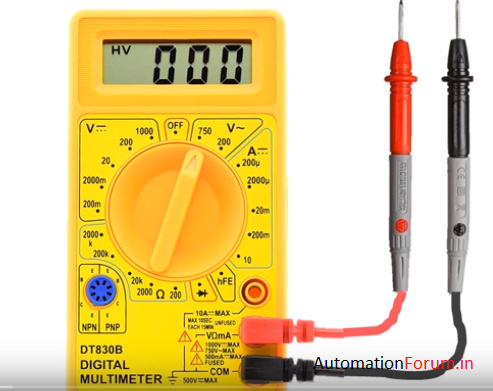# How to use a mutimeter?

## What is a mutimeter?

A mutimeter or mutitester also known as VOM (volt-ohm-milliammeter). It is used as a voltmeter or ohmmeter or as a ammeter.

It is used to measure electrical values like voltage, resistance and current. It is an electronic measuring instrumentthat combines several measurement functions in one unit.• A multimeter can be a handy device useful for finding basic faults and field service jobs, or a bench instrument that can measure with a high degree of accuracy. They can be used to solve electrical problems in a wide range of domestic and industrial devices, such as electronic equipment, motor controls, appliances, power supplies and wiring systems.

• A multimeter allows you to understand what is going on in your circuits. Whenever something in your circuit isn’t working, the multimeter will help you troubleshooting.

## A multimeter is composed by four essential sections:

Display: this is where the measurements are displayed

Selection knob: this selects what you want to measure

Ports: this is where you plug in the probes

Probes: a multimeter comes with two probes. Generally one is red and the other is black.

## To measure voltage:

1. Set the mode to V with a wavy line if you’re measuring AC voltage or to the V with a straight line if you’re measuring DC voltage.

2. Make sure the red probe is connected to the port with a V next to it.

3. Connect the red probe to the positive side of your component, which is where the current is coming from.

4. Connect the COM probe to the other side of your component.

5. Read the value on the display.

## Let’s see an example on how to measure the voltage drop across a resistor

• In order to measure the voltage, we create a circuit by applying 5v to the led and resistor connected in series.

• Plug the probes into the port labeled common and voltage. Generally, black means common and red means positive.

• Then adjust the knob for DC voltage in the range we expect that is between 0to 5 volts. So adjust the knob with a maximum of 20V since 2V is too low.

• With the circuit ‘ON’ touch the positive probe to the resistor connecting to the led and the common probe to the resistor lead connecting to the power supply negative terminal.

• As shown in the image, you can see 3V drop across the resistor. If you accidentally switch negative and positive probes, you will see negative voltage in the display.

## How does continuity work?

### Continuity = almost 0 ohms

• If there is a very low resistance between two points, which is less than a few ohms, the two points are electrically connected and a continuous sound will be heard.

• If the sound is not continuous or you do not hear any sound, it means that what you are testing has a bad connection or is not connected at all.

• To test continuity, you must turn off the system. Disconnect the power supply.

• Touch the two probes together and, when connected, you will hear a continuous sound.

• To test the continuity of a cable, you only need to connect each probe to the cable tips.

## Lets see few examples on how multimeter works.

1. RESISTANCE measuring

With this range we can measure upto 2k ohm. We see here 39 ohm but the range 200 ohm will add a significant digit.

1. DC VOLTAGE measuring

With this range, we can measure up to 20 V.

This is the range that gives the best accuracy for the example (3 significant digits).

Here we read : U = 4.27 V

1. CURRENT measuring

With this range, we can measure current up to 200 mA. If the intensity is higher, the AMMETER MAY BE DAMAGED ! We must begin the highest range (10 A) to get an idea of the value of the measurement.

4.Measure the RMS (effective) value of ALTERNATING VOLTAGES

With this range, we can measure up to 200 V.

Multimeter Simulator

http://www.physics-chemistry-interactive-flash-animation.com/electricity_electromagnetism_interactive/multimeter.htm